# What is Area?

Area is the size of a surface!

### Example:

These shapes all have the same area of 9: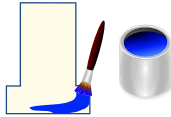It helps to imagine how much paint would cover the shape.

## Area of Simple Shapes

There are special formulas for certain shapes:

### Example: What is the area of this rectangle?The formula is:

Area = w × h
w = width
h = height

The width is 5, and the height is 3, so we know w = 5 and h = 3:

Area = 5 × 3 = 15

## Area by Counting Squares

We can also put the shape on a grid and count the number of squares:The rectangle has an area of 15

Example: When each square is 1 meter on a side, then the area is 15 m2 (15 square meters)

## Square Meter vs Meter Square

The basic unit of area in the metric system is the square meter, which is a square that has 1 meter on each side:1 square meter

Be careful to say "square meters" not "meters squared":There are also "square mm", "square cm" etc, learn more at Metric Area.## Approximate Area by Counting Squares

Sometimes the squares don't match the shape exactly, but we can get an "approximate" answer.

#### One way is:

• more than half a square counts as 1
• less than half a square counts as 0

Like this: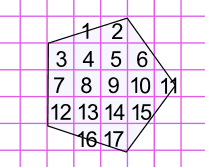This pentagon has an area of approximately 17

#### Or we can count one square when the areas seem to add up.

Example: Here the area marked "4" seems equal to about 1 whole square (also for "8"):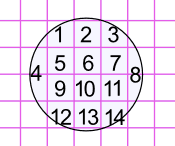This circle has an area of approximately 14

### Example: The circle has a radius of 2.1 meters: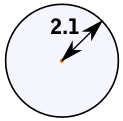The formula is:

Area = π × r2

Where:

Area = 3.1416... × (2.1m)2
= 3.1416... × (2.1m × 2.1m)
= 13.854... m2

So the circle has an area of 13.85 square meters (to 2 decimal places)

## Area of Difficult Shapes

We can sometimes break a shape up into two or more simpler shapes:

### Example: What is the area of this Shape?Let's break the area into two parts: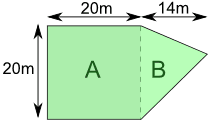Part A is a square:

Area of A = a2 = 20m × 20m = 400m2

Part B is a triangle. Viewed sideways it has a base of 20m and a height of 14m.

Area of B = ½b × h = ½ × 20m × 14m = 140m2

So the total area is:

Area = Area of A + Area of B

Area = 400m2 + 140m2

Area = 540m2

## Area by Adding Up Triangles

We can also break up a shape into triangles:Then measure the base (b) and height (h) of each triangle: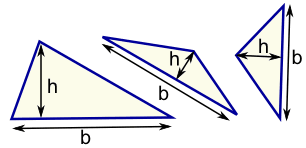Then calculate each area (using Area = ½b × h) and add them all up.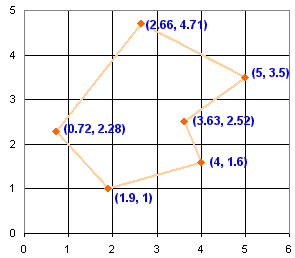## Area by Coordinates

When we know the coordinates of each corner point we can use the Area of Irregular Polygons method.

There is an Area of a Polygon by Drawing Tool that can help too.